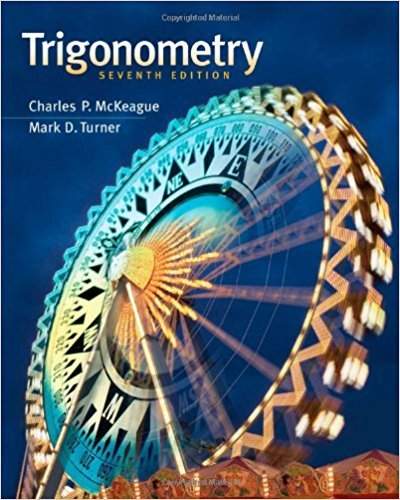×
Log in to StudySoup
Get Full Access to Trigonometry - 7 Edition - Chapter 4.3 - Problem Problem 4
Join StudySoup for FREE
Get Full Access to Trigonometry - 7 Edition - Chapter 4.3 - Problem Problem 4

Already have an account? Login here
×
Reset your password

# Graph one cycle of y sin x 2 3ISBN: 9781111826857 186

## Solution for problem Problem 4 Chapter 4.3

Trigonometry | 7th Edition

• Textbook Solutions
• 2901 Step-by-step solutions solved by professors and subject experts
• Get 24/7 help from StudySoup virtual teaching assistantsTrigonometry | 7th Edition

4 5 1 394 Reviews
18
1
Problem Problem 4

Graph one cycle of y sin x 2 3

Step-by-Step Solution:
Step 1 of 3

Zane Ghali Calculus 1 Notes Area Under A Curve Area Under A Curve Notes ● The area under the curve of a function is called an integral, and gives us a new equation. The integral is the opposite of a derivative. ● There are 3 Methods for finding the area under a function. The 3 methods involve creating a rectangle extending from the x-axis of a graph, all the way up to the curve, meeting it at certain points based on the kind of rectangle. The 3 types of rectangles are: Right-end rectangles, left-end rectangles, and midpoint rectangles. ● To find the area underneath the graph of “f(x)=2x+2” we need to first determine what kind of rectangle we want to use, and what our bounds are for the x-axis of our function.

Step 2 of 3

Step 3 of 3

##### ISBN: 9781111826857

Unlock Textbook Solution

Enter your email below to unlock your verified solution to:

Graph one cycle of y sin x 2 3